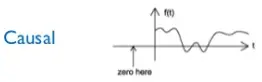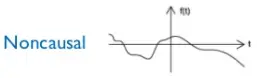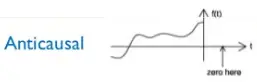# Causal, Non-Causal & Anti-Causal Signals

Causal Signal

A signal that does not start before t=0 is a causal signal i.e. x(t)=0, ∀ t<0.

Note: A system is causal or non-anticipatory if the output at any time to depends only on the values of the input at the present time and in the past.Note: All memoryless systems are causal systems since the output responds only to the current value of the input.

Non-Causal Signal

A signal that starts before t=0 is a non-causal signal.Anti-Causal Signal

A signal that ends after t=0 is an anti-causal signal i.e. x(t)=0, ∀ t>0.Note: Any signal x(t) that does not contain any singularities (a delta function or its derivative) at t=0 can be written as the sum of a causal part x+(t) and anti-causal part x(t)  i.e.

x(t) = x+(t) +  x(t)

For example, x(t) = e−at  can be written as

x(t) = e−at u(t) + e−at u(−t)

causal       anti-causal

Note: Multiplying the signal by the unit step ensure that the resulting signal is causal.

error: Content is protected !!